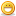1. ##Programming Logic Help

Ok this is a problem for my Programming Logic class that I need help with

The members of the board of a small university are considering voting for a pay increase for their
25 faculty members. They are considring a pay increase of 8%. How ever before doing so, they want to know how much this pay increase will cost Design an algorithm that will prompt for and accept the current salary for each of the faculty members, then calculate and display their individual pay increases. At the end of the algorithm, print the total faculty payroll before and after the pay increase, and the total pay increase involved.

Better Version.

Inputs
member_salary

Processing
calculate member_new_salary (member_salary + 8%) or (member_salary * 1.08)
calculate member_pay_increase (member_new_salary  member_salary)
calculate total_current_salaries (sum of all member_salary)
calculate total_new_salaries (sum of all member_new_salary)

Outputs
display member_pay_increase
display total_current_salaries
display total_new_salaries
display total_pay_increases (total_new_salaries - total_current_salaries)

Pseudocode

Prompt for member_ original_salary
Get Salary
DoWhile salary = 25
Display "Members Original Salary"; member_original_salary
EndDo

Calculate total_current_salary
Get Salary
DoWhile salary = 25
Calculate total_current_salary = member_original_salary + 25 member_salary
Display "Total of Current Salaries"; total_current_salary
EndDo

Calculate member_new_salary
Prompt for original salary
Get Salary
DoWhile salary = 25
Calculate member_new_salary = member_salary * 8%
Display "New Salary"; member_new_salary
EndDo

Calculate member_pay_increase
Prompt for member_salary
Get Salary
DoWhile salary = 25
calculate member_pay_increase = member_new_salary - member_salary
Display "Pay Increase"; member_pay_increase
EndDo

Calculate total_current_salaries
Prompt for member_salary
Get Salary
calculate total_current_salaries = total_current_ salaries + 25 member_salary
Display "Total Current Salaries"; = total_current_salaries
end

Calculate total_new_salaries
Prompt for member_salary
Get Salary
Calculate total_new_salaries = total_current_salaries + member_pay_increase
Display "Total of new Salaries"; = total_new_salaries
end

I just need to know if it looks right, It also might help to look at it in word pad. But I need to know if my Pseudocode look right.2. ##hmmmmmm... not sure what you're trying to do with that algorithm. I had something a little more like this in mind. I'm just going to punch out the mainline algorithm in C++, so if you want the pseudocode, let me know.

#include <iostream>
#include <cstdlib>

using namespace std;

double calcEmp();
const int EmployeeNum = 25;

int main()
{
double totalInc=0;
for(counter=0;counter<EmployeeNum;counter++)
{
totalInc += calcEmp();
}

cout << "The final payroll for all 25 employees is: " << totalInc;
return 0;
}

double calcEmp()
{
double empPay, finalPay;
cout << "Please enter the employee's pay in dollar amt: ";
cin >> empPay;
finalPay = empPay+2*empPay;
return(finalPay);
}

yeah... somethin like that. Now if only I could do my OWN homework!! hahahaha.3. ##Ok let me explain

Ok I'm in a Logic Class for school we get problems out of the book. The one I posted is in the book. What the teacher is trying to teach us is not programming but the Logic behind the program. These are the first steps to creating a program, I know I never used this **** when I was programming but it does have it's points.

Inputs= what the person needs to enter into the program for it to work

Processing= ok it's different then C++ code, you make a plan basically the steps that your program will make in order for it to run.

Outputs= is what the program shows to the user, for example display, print **** like that.

Psuedocode - is a set of instructions or procedure that are word/code statments for example I use total_current_salary. It's like a scientific procedure, or a recipe for creating a meal.

Now he want's us to create the inputs,outputs, and processing steps and the psuedocode. I wanted some help to see if it was correct.4. ##If you still need help or understand it I can try to be more specific.####Posting Permissions

• You may not post new threads
• You may not post replies
• You may not post attachments
• You may not edit your posts
•